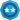Choose a Product Community
Resources

## SOAP UI: Assert JSON response parameter with 2 possible values

SOLVEDFrequent Contributor

## SOAP UI: Assert JSON response parameter with 2 possible values

Hi

I have use case where in response, I am getting code as a parameter where possible values may be 110 and 200

I applied Script assertion as below

``````import groovy.json.*
def response = context.expand( '\${Hit SMS Request#Response}' )

def parsed = new JsonSlurper().parseText(response)

def smscode = parsed.code.toString()

if (smscode=="110" || "200")
{
log.info "Pass"

}
else
{
log.info "Fail"

}
assert smscode.contains('110' || '200')``````

2 REPLIES 2Champion Level 2

Hi,

Assuming that smscode actually is value from the response you're searching for, then the 'if' needs to be modified.

You could try a log.info on smscode before the 'if' to check.

The If should look like....

``if ((smscode=="110") || (smscode=="200"))``

The string.contains method only accepts a single string, not an expression.

You could turn this around and do something like....

``assert '110,200'.contains(smscode);``

Or, seeing as you have used the IF to check the string already, assign the result of the IF and assert that, e.g.

``````def result = '';

if ((smscode=="110") || (smscode=="200"))
{
result = "Pass";
}
else
{
result = "Fail";
}

log.info("Result is \${result}.");

// Assert the result from the IF.
assert result == "Pass";``````Frequent Contributorcancel
Showing results for